# AP Board 5th Class Maths Solutions 6th Lesson Geometry

Andhra Pradesh AP Board 5th Class Maths Solutions 6th Lesson Geometry Textbook Exercise Questions and Answers.

## AP State Syllabus 5th Class Maths Solutions Chapter 6 Geometry

Do these: (TextBook Page No.87)

Question 1.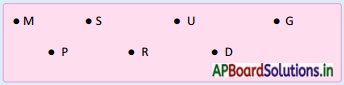Point – M, Point – S, Point – U, Point – G, Point – P, Point – R, Point – D.

Question 2.
Write any 5 distinct points from the above.
Point – A, Point – B, Point – C, Point – E, Point – F.

Question 3.
Note down any 3 points in your note book and name them.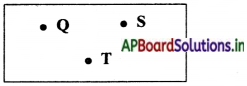Point – Q, Point – S, Point – T.Do this: (TextBook Page No. 88)

Question 1.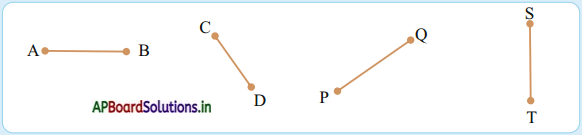Line segment – AB, line segment – CD, line segment – PQ, line segment – ST.

Question 2.
Draw some line segments by joining following points and name them.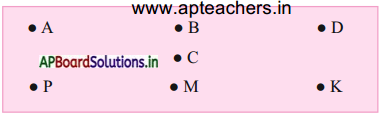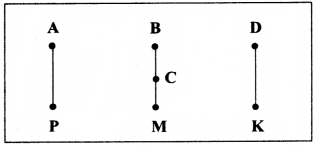Line segment $$\overline{\mathrm{AB}}$$
Line segment $$\overline{\mathrm{BC}}$$
Line segment $$\overline{\mathrm{CM}}$$
Line segment $$\overline{\mathrm{DK}}$$

Question 3.
Find the line segments in the figures given below.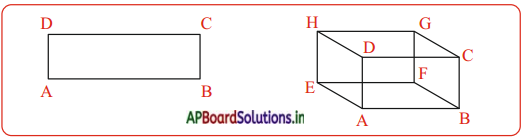Fig (i): $$\overline{\mathrm{AB}}$$, $$\overline{\mathrm{BC}}$$, $$\overline{\mathrm{CD}}$$, $$\overline{\mathrm{DA}}$$
Fig (ii): $$\overline{\mathrm{AB}}$$, $$\overline{\mathrm{BC}}$$, $$\overline{\mathrm{CD}}$$, $$\overline{\mathrm{DA}}$$, $$\overline{\mathrm{EF}}$$, $$\overline{\mathrm{GH}}$$, $$\overline{\mathrm{FG}}$$, $$\overline{\mathrm{EH}}$$, $$\overline{\mathrm{AE}}$$, $$\overline{\mathrm{BF}}$$, $$\overline{\mathrm{CG}}$$, and $$\overline{\mathrm{DH}}$$.Do this: (TextBook Page No. 90)

Observe the following rays and name them.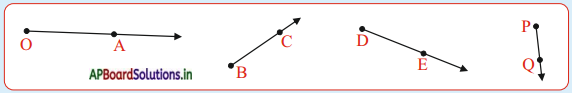OA ray, BC ray, DE ray and PQ ray.

Do this: (TextBook Page No. 91)

Question 1.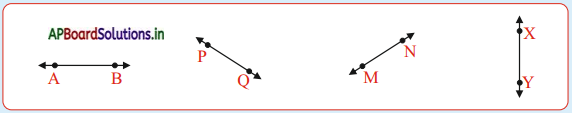Line AB, Line PQ, Line MN, Line XY.

Question 2.
Take any two points and draw a line.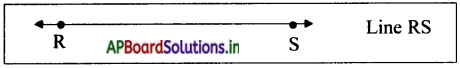Exercise 1:

Question 1.
Take any six points on a paper and name them.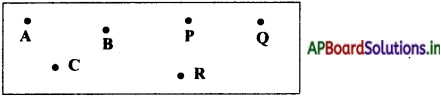Question 2.
Join the points given below. Name the line segments so formed in the figures.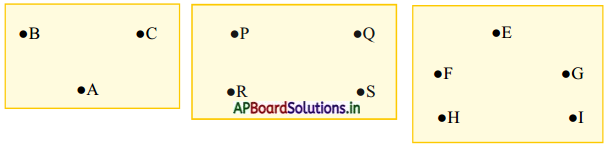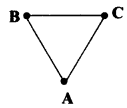Line segment — AB
Line segment — AC
Line segment — BC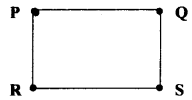Line segment — PQ
Line segment — PR
Line segment — RS
Line segment — QS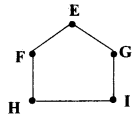Line segment — EF
Line segment — EG
Line segment — FH
Line segment — GI
Line segment — HI.Question 3.
Classify the following as ray, line and line segment. Also name them.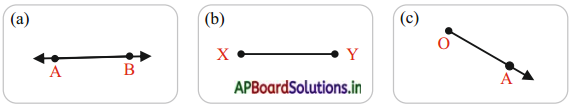a) Line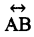b) Line segment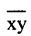c) Ray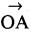Question 4.
Check whether the statements are True (T) or False (F). If it is false, give the reason.

a) A ray has a definite length. ( )
F

b) A ray has one end point. ( )
T

c) A line segment has a fixed length. ( )
T

d) A line segment has no end points. ( )
F

e) A line has no end points. ( )
TQuestion 5.
Represent the rays from the figure given below.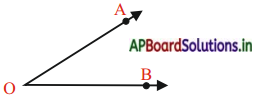From figure, Ray –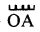and Ray –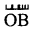.

Question 6.
Find rays, lines and line segments from the figure given below.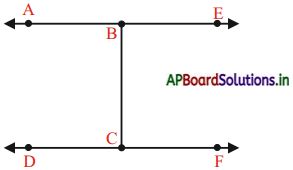Rays : $$\overrightarrow{\mathrm{BA}}$$, $$\overrightarrow{\mathrm{BE}}$$, $$\overrightarrow{\mathrm{CD}}$$, $$\overrightarrow{\mathrm{CF}}$$
Lines : $$\overleftrightarrow{\mathrm{AE}}$$, $$\overleftrightarrow{\mathrm{DF}}$$
Line segment: $$\overline{\mathrm{BC}}$$

Question 7.
How many straight lines can be drawn through ?
a) One point
b) Two distinct points.
a) From one point many straight lines can be possible to draw.
b) From two distinct points only one line can be possible to draw.

Question 8.
a) Write any three points.
b) Write any Two rays.
c) Write any five line segments.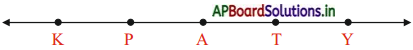a) Point – K, Point – P, Point – A
b) Ray – $$\overleftrightarrow{\mathrm{KY}}$$, Ray – $$\overleftrightarrow{\mathrm{AT}}$$
c) Line segments : $$\overline{\mathrm{KP}}$$, $$\overline{\mathrm{PA}}$$, $$\overline{\mathrm{AT}}$$, $$\overline{\mathrm{TY}}$$ and $$\overline{\mathrm{KA}}$$Do these: (TextBook Page No. 94)

Question 1.
Observe the adjacent figure and fill in the blanks.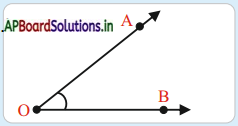a) Vertex is …………….
O

b) Arms are ……………
$$\overrightarrow{\mathrm{OA}}$$ and $$\overrightarrow{\mathrm{OB}}$$

c) Angle is ……………..
∠AOB

Question 2.
Observe the various items in your classroom and write the things where angles occur.
Corners of black board.
Corners of table.
Corners of book.
Edges of room door etc.

Do this: (TextBook Page No.94)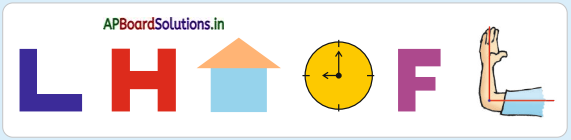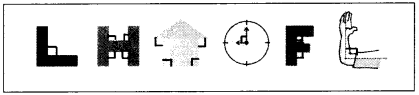Do this: (TextBook Page No.95)

Question 1.
Observe the angles given below and classify them as acute, obtuse and right angles.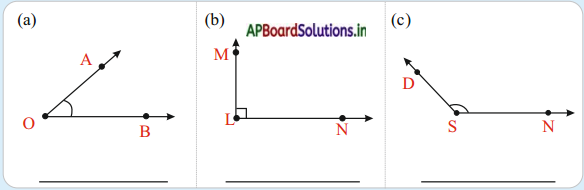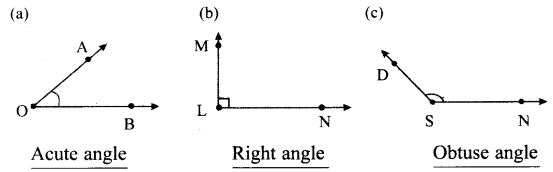Do this: (TextBook Page No.97)

Classify the angles given below.
25°, 30°, 45°, 120°, 150°, 90°, 160°, 95°, 100°, 60°, 80°, 75°, 110°

Acute angles : _____________
25°, 30°, 45°, 60°, 75° and 80°.

Right angle: ______________
90°

Obtuse angles: _____________
95°, 100°, 110°, 120°, 150°.Exercise 2:

Question 1.
Observe the following angles and write them.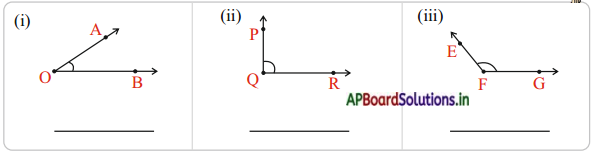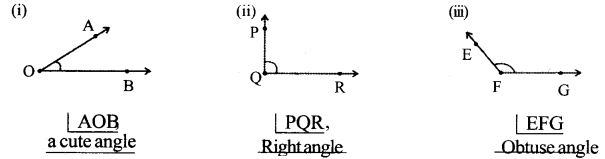Question 2.
Observe the following. Name the type of angle.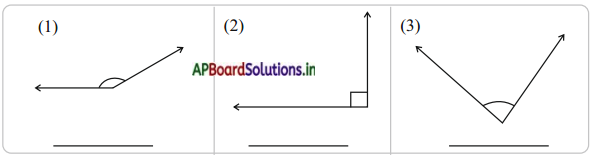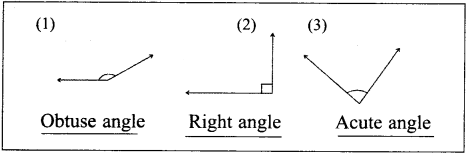Question 3.
Observe the picture given below. Count the number of acute angles, obtuse angles and right angles.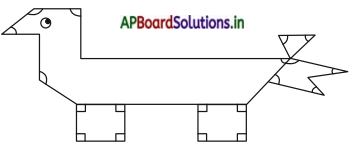No. of Right angles ___________
No. ofAcute angles ___________
No. of Obtuse angles ___________
No. of Right angles 10
No. of Acute angles 10
No. of Obtuse angles 4.Exercise 3:

Question 1.
State what figures are open and which are closed.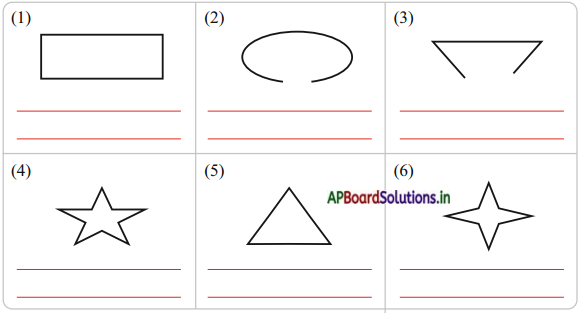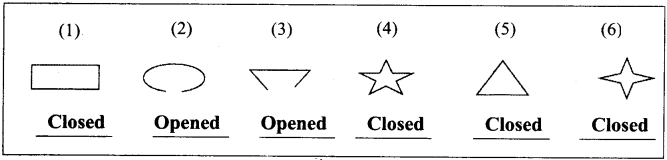Question 2.
Draw three simple closed figures by using straight lines only.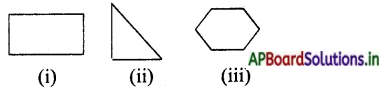Question 3.
Draw three simple closed figures by using both straight lines and curved lines.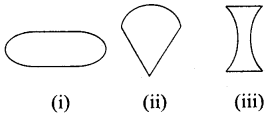Question 4.
What is angle in a rectangle?
A rectangle has right angle in its corners.

Question 5.
Square is a special case of rectangle.
What is its speciality in terms of sides?
In square all sides are equal in lengths.

Question 6.
Why there are four angles in a rectangle?
In a rectangle four line segments are joined at corners. So, it has four angles.

Question 7.
What are the properties of a rect angle?
In a rectangle, opposite sides are equal in Lengths. Each angle is right angle.

Question 8.
Give some examples of articles in a square shape.
Place of chalk box, Dice, Cube etc.Do this: (TextBook Page No.103)

Question 1.
Here are some letters. Tick the letters which look different in the mirror.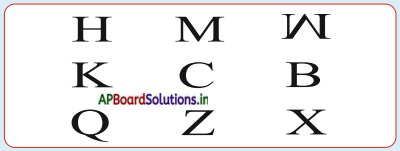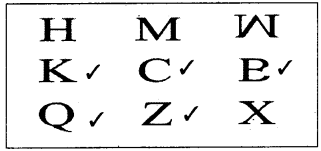Do this: (TextBook Page No.104)

Question 1.
Put (✓) mark to the picture which have line of symmetry.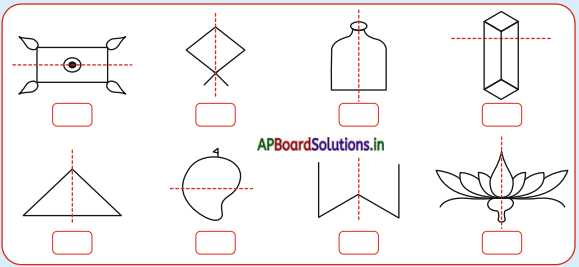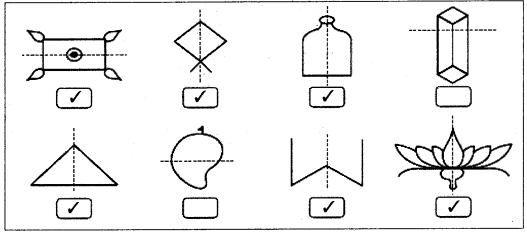Question 2.
Draw possible number of symmetrical lines for the given figures.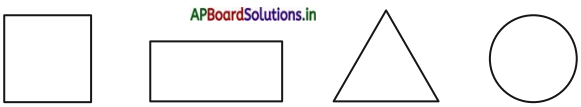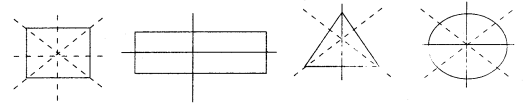Complete the following table.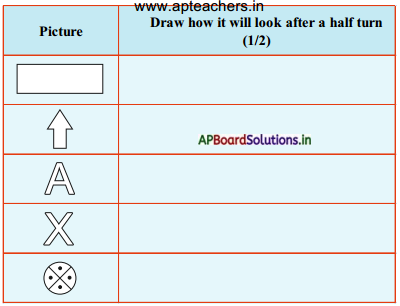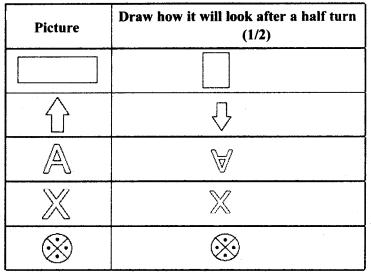Do this: (TextBook Page No.107)

Complete the following table.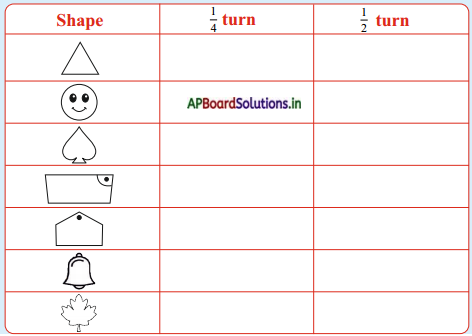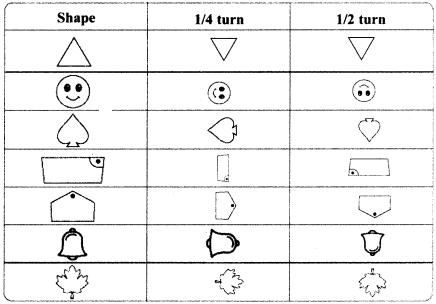Exercise 4:

Question 1.
Match each letter with its mirror image. The dotted line with every letter shows the mirror.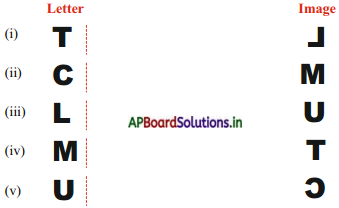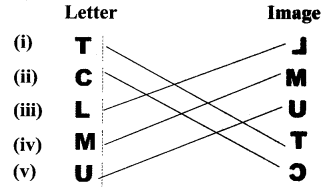Question 2.
Check whether the dotted line represents the line of symmetry or not and put a tick mark under the figure with line of symmetry.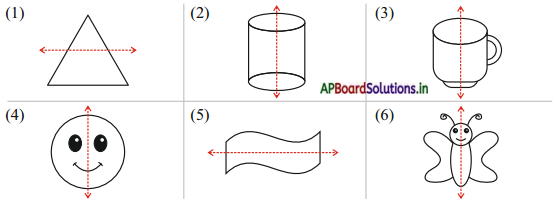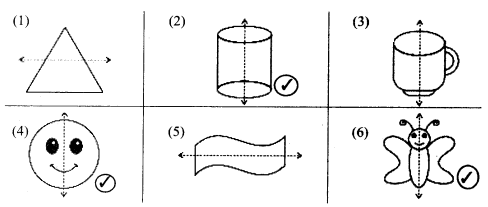Question 3.
Draw possible lines of symmetry for the figures given below, wherever possible.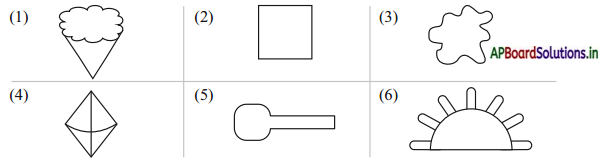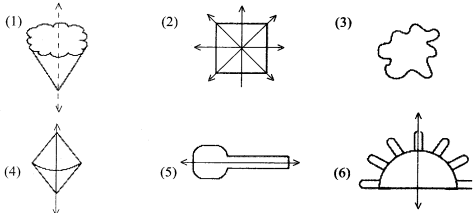Question 4.
Complete the following table.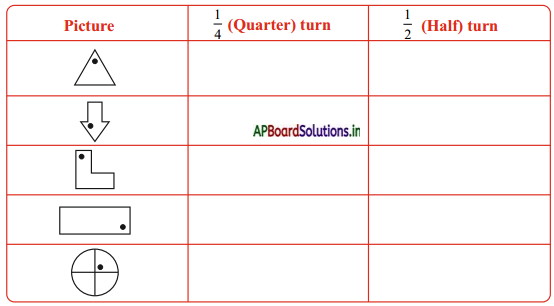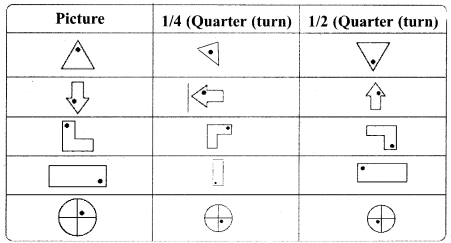Try these: (TextBook Page No.109)

Question 1.
Arrange some patterns using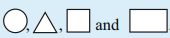.Question 2.
What should come next?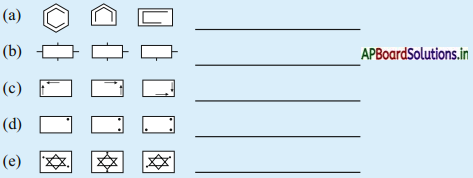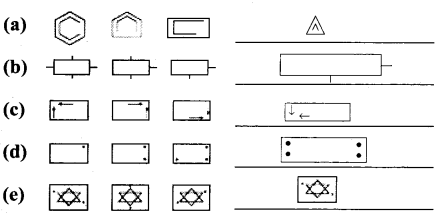Do this: (TextBook Page No.110)

Question 1.
Find the perimeter of the following.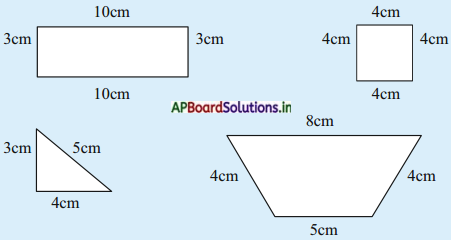i) Perimeter of given figure = 10 cm + 3 cm + 10 cm + 3 cm = 26 cm
ii) Perimeter of given figure = 4 cm + 4 cm + 4 cm + 4 cm = 16 cm
iii) Perimeter of given figure = 3 cm + 5 cm + 4 cm = 12 cm
iv) Perimeter of given figure = 8 cm + 4 cm + 5 cm + 4 cm = 21 cm.

Question 2.
Find the perimeter of the following rectangles.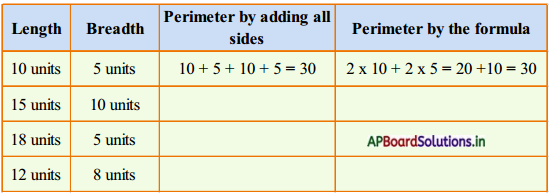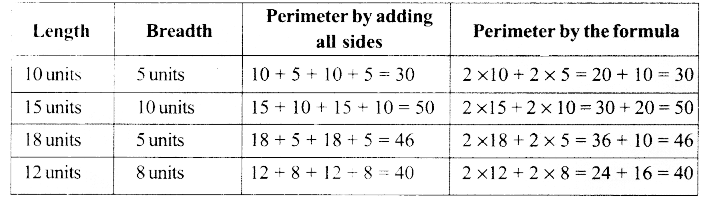Do these: (TextBook Page No.112)

Question 1.
Find the perimeter of a square with a side of 3 ems.
Length of side of square 3 cm
Perimeter of a square = 4 × 3 cm = 12 cm.

Question 2.
Find the perimeter of a square with a side of 12 ems.
Length of side of square = 12 cm
Perimeter of a square 4 × 12 = 48 cm.

Exercise 5:

Question 1.
Find the perimeter of a rectangular field whose length is 40 m and breadth is 25 m.
Length of rectangular field l = 40 cm
Breadth of rectangular field b = 25 m
Perimeter of rectangular field = P
= 2l + b
= 2 × 40 + 2 × 25
= 80 + 50 = 130 m.

Question 2.
Find the perimeter of a park in square shape side with 25m.
Side of square = 25 m
Perimeter of a square = 4 × 25 = 100 mQuestion 3.
Find the area of a square whose one side is 13 cms.
Side of square = 13 cm
Area of a square = 13 × 13 = 169 sq cm

Question 4.
Find the area of a black board with a length of 240 cms and breadth 120 cms.
Length of black board = 240 cm
Breadth of black board = 120 cm
Area of black board = 240 × 120 = 28,800 sq cm.

Question 5.
Find the cost of fencing to a square park whose side is 200 m. Cost for lm is ^ 30.